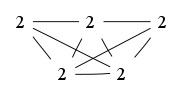# The Markov Bases Database

## BPg_bin

The binary graphical model of the bipyramid graph.

It is a hierarchical model of 5 variables. The dimension of the model is 23.The cardinality of the statespace is 32.

### Properties of the Markov basis

 Markov degree 2 8

 degree 2 8

The model has the following properties:

• All variables are binary.
• It is a graphical model.
• The minimal Markov basis is unique.
• The semigroup is normal.### FILES

 Markov basis: sufficient statistics matrix: BPg_bin.mar (781 b) BPg_bin.mat (2.04 kb) BPg_bin.mod (36 b) BPg_bin.tar.gz (4.74 kb)